##### Actions

(diff) ← Older revision | Latest revision (diff) | Newer revision → (diff)

The partial differential equation that can be written in complex form as follows: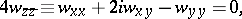where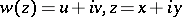, and that can be reduced to the elliptic system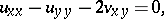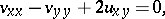in the real independent variablesand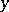. The homogeneous Dirichlet problem in a disc: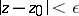, where the radiusis as small as one pleases, for the Bitsadze equation has an infinite number of linearly independent solutions . The Dirichlet problem for the inhomogeneous equation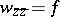in the discis normally solvable according to Hausdorff, since it is neither a Fredholm problem nor Noetherian; in a bounded domain containing a segment of the straight line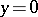, this problem is not even a Hausdorff problem, even though the homogeneous problem has only one zero solution .

How to Cite This Entry: